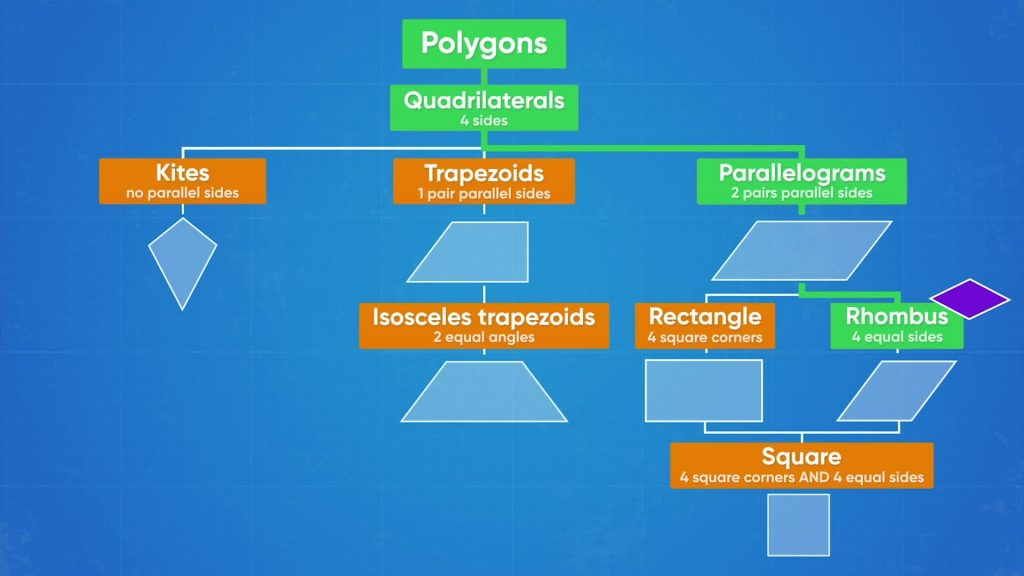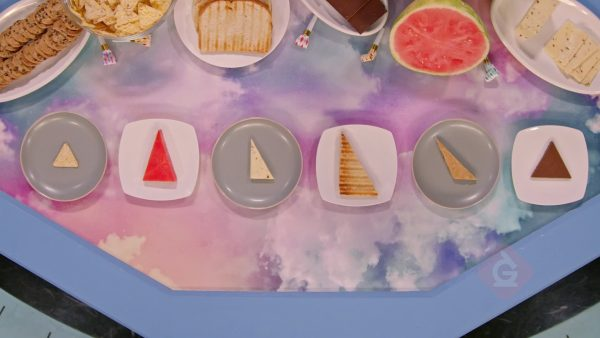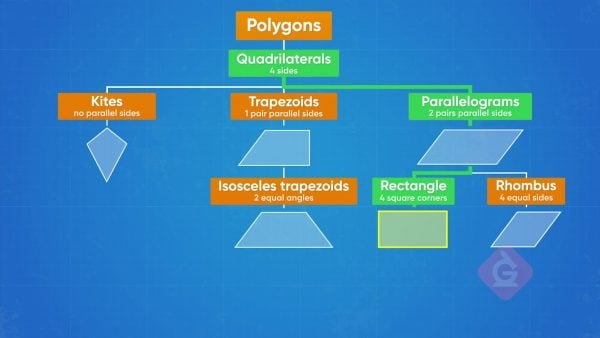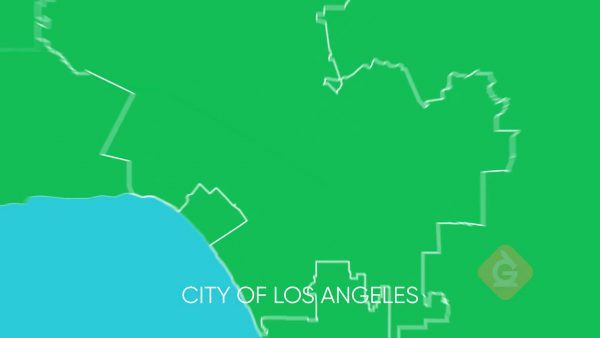Classify Shapes in a Hierarchy | Math Lesson For Kids | Grades 3-5
1%
It was processed successfully!WHAT IS CLASSIFYING SHAPES IN A HIERARCHY (QUADRILATERALS & TRIANGLES)

What are the properties of shapes? 2D shapes are flat and can hold area. When all the sides are straight, the shape is a polygon. These shapes have properties like side lengths and angles.

To better understand classifying shapes in a hierarchy (quadrilaterals & triangles)…

WHAT IS CLASSIFYING SHAPES IN A HIERARCHY (QUADRILATERALS & TRIANGLES). What are the properties of shapes? 2D shapes are flat and can hold area. When all the sides are straight, the shape is a polygon. These shapes have properties like side lengths and angles. To better understand classifying shapes in a hierarchy (quadrilaterals & triangles)…

## LET’S BREAK IT DOWN!

### Triangle PartyWe are having a Triangle Party! We have many familiar objects, all with three straight sides. One is a chip with all equal length sides. Another is a sail for our model sailboat. It has three different side lengths and one 90° angle. We know that triangles can have acute angles, meaning angles smaller than 90°, obtuse angles that are bigger than 90°, or right angles that are equal to 90°. When the sides of a triangle are all the same length, it is equilateral. If only two are the same length, it is isosceles, and if all the lengths are different, it’s scalene. What kind of triangles are our objects? The chip is an equilateral triangle because of its three equal sides. The sail is a right scalene triangle because all the lengths are different, and it has a 90° angle. Try this yourself: What kind of triangle is a coat hanger if it has two equal-length sides and one angle larger than 90°?

Triangle Party We are having a Triangle Party! We have many familiar objects, all with three straight sides. One is a chip with all equal length sides. Another is a sail for our model sailboat. It has three different side lengths and one 90° angle. We know that triangles can have acute angles, meaning angles smaller than 90°, obtuse angles that are bigger than 90°, or right angles that are equal to 90°. When the sides of a triangle are all the same length, it is equilateral. If only two are the same length, it is isosceles, and if all the lengths are different, it’s scalene. What kind of triangles are our objects? The chip is an equilateral triangle because of its three equal sides. The sail is a right scalene triangle because all the lengths are different, and it has a 90° angle. Try this yourself: What kind of triangle is a coat hanger if it has two equal-length sides and one angle larger than 90°?

### Hanging PicturesWe are in art class and need to hang pictures in their matching frames with the same shape. All the frames are sorted into their most specific category of 2D shape, but we still need to sort the pictures. All the frames have four straight sides, so we know they are all quadrilaterals. Some frames have one set of parallel sides; these are trapezoids. Others have two sets of parallel sides; these are parallelograms. Some of the parallelograms have right angles, which means they are rectangles. Your friend points to a pile of frames that are parallelograms that have four equal sides but no right angles. Those are rhombi, or a rhombus if we only have one. The last pile is even more specific, with 4 right angles and 4 equal sides. Those are squares! Your first picture has four straight sides, two pairs of parallel sides with different lengths. That means the most specific category we can choose is a parallelogram. That is where we will find the matching frame! Try this yourself: There is a picture with four equal sides and no right angles. What is the most specific category of shape for the picture?

Hanging Pictures We are in art class and need to hang pictures in their matching frames with the same shape. All the frames are sorted into their most specific category of 2D shape, but we still need to sort the pictures. All the frames have four straight sides, so we know they are all quadrilaterals. Some frames have one set of parallel sides; these are trapezoids. Others have two sets of parallel sides; these are parallelograms. Some of the parallelograms have right angles, which means they are rectangles. Your friend points to a pile of frames that are parallelograms that have four equal sides but no right angles. Those are rhombi, or a rhombus if we only have one. The last pile is even more specific, with 4 right angles and 4 equal sides. Those are squares! Your first picture has four straight sides, two pairs of parallel sides with different lengths. That means the most specific category we can choose is a parallelogram. That is where we will find the matching frame! Try this yourself: There is a picture with four equal sides and no right angles. What is the most specific category of shape for the picture?

### Packing for Summer CampSuppose you are packing for summer camp and decide to sort your belongings by shape. You have a book, a clothes hanger, and a kite. Looking at the shapes, you notice they all have straight edges, so you put them all in the polygon pile. The hanger has three sides, so you know it is a triangle. The book and kite both have four sides, so they are quadrilaterals. The book has all right angles, so it is also a rectangle. But what shape is the kite? It has straight sides, so it is a polygon. The kite has four sides, so it is also a quadrilateral. Even more specifically, it has two pairs of adjacent sides that are equal in length, which makes it a kite! Try this yourself: What are all the categories that a shape with four equal-length sides, no right angles, and no parallel sides could belong to?

Packing for Summer Camp Suppose you are packing for summer camp and decide to sort your belongings by shape. You have a book, a clothes hanger, and a kite. Looking at the shapes, you notice they all have straight edges, so you put them all in the polygon pile. The hanger has three sides, so you know it is a triangle. The book and kite both have four sides, so they are quadrilaterals. The book has all right angles, so it is also a rectangle. But what shape is the kite? It has straight sides, so it is a polygon. The kite has four sides, so it is also a quadrilateral. Even more specifically, it has two pairs of adjacent sides that are equal in length, which makes it a kite! Try this yourself: What are all the categories that a shape with four equal-length sides, no right angles, and no parallel sides could belong to?

### Using a HierarchySasha and Derek are trying to explain their location to a friend. Sasha says they are in Los Angeles, but Derek says they are in California. How do we know who is right? They both are! We can understand this using a hierarchy. At the highest level, they are in California. More specifically, they are in the city of Los Angeles. Even more specifically, they are in Hollywood! So, knowing that someone is in Hollywood, we also know that they are in Los Angeles and California because those are the levels above Hollywood in our location hierarchy. Try this yourself: Think about your school. What is its location hierarchy?

Using a Hierarchy Sasha and Derek are trying to explain their location to a friend. Sasha says they are in Los Angeles, but Derek says they are in California. How do we know who is right? They both are! We can understand this using a hierarchy. At the highest level, they are in California. More specifically, they are in the city of Los Angeles. Even more specifically, they are in Hollywood! So, knowing that someone is in Hollywood, we also know that they are in Los Angeles and California because those are the levels above Hollywood in our location hierarchy. Try this yourself: Think about your school. What is its location hierarchy?

## CLASSIFY SHAPES IN A HIERARCHY (QUADRILATERALS & TRIANGLES) VOCABULARY

Property
A characteristic that is true of a geometric shape in a given category.
Category
A classification of shapes with a defined set of properties.
Polygon
A closed shape with all straight sides.
Angle
A measurement of the rotation between two lines with a common endpoint.
Right Angle
An angle that measures 90°.
Acute Angle
An angle that measures between 0° and 90°.
An angle that measures between 90° and 180°.
A three-sided polygon.
A triangle where all sides have an equal length.
A triangle with two equal sides.
A triangle with no sides the same length.
A four-sided polygon.
A quadrilateral with two sets of parallel sides.
A categorization method where things are sorted into more and more specific groups, and the subgroups retain all the properties of the supergroup.
A group that can be divided into smaller subgroups based on a property of the items you are sorting.
A group with a more specific property than the supergroup. For example, of the supergroup “all US states,” there is a subgroup “states on the West coast.”
A parallelogram with all right angles.
A quadrilateral with 4 equal sides and two pairs of parallel sides.
A quadrilateral with at least one set of parallel sides.
A quadrilateral with exactly one pair of parallel sides.
A five-sided polygon.
A six-sided polygon.
A seven-sided polygon.
An eight-sided polygon.
A nine-sided polygon.

## CLASSIFY SHAPES IN A HIERARCHY (QUADRILATERALS & TRIANGLES) DISCUSSION QUESTIONS

### What does it mean for parallelogram to be a subgroup of quadrilateral?

It means that a parallelogram has all the properties of a quadrilateral, plus the properties specific to parallelograms.

### Why is a square both a parallelogram and a kite?

The square inherits the properties of the kite (two sets of adjacent sides with equal length) through the rhombus (four equal sides), and the properties of the parallelogram (two sets of parallel sides) through the rectangle (all right angles). Because the square keeps the properties that define its supergroups, a square is also a parallelogram and a kite.

### Can a triangle be a quadrilateral? Explain.

No. A triangle and a quadrilateral are both classified on the property of “number of sides.” A triangle is a shape with exactly three sides, and a quadrilateral is a shape with exactly four sides. This means that a shape cannot be both a triangle and a quadrilateral.

### Is a parallelogram a trapezoid? Explain

(Exclusive definition) No. A trapezoid is a shape with exactly one set of parallel sides, so a parallelogram cannot be a trapezoid. (Inclusive definition) Yes. A trapezoid is a shape with at least one set of parallel sides, so parallelogram is a subgroup of trapezoid.

### How would you define a hierarchy?

A hierarchy is a system of classification based on sets of properties that become more and more specific as you move down the hierarchy. All subgroups retain all the properties of their supergroups, so in geometry, shapes inherit the properties of the categories above them in the hierarchy.
X

## Success

We’ve sent you an email with instructions how to reset your password.
Ok